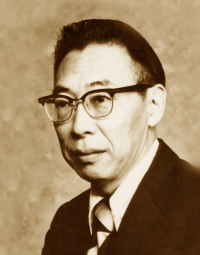Prof. Ven Te Chow
(1919-1981)

 Chow, Froude, and Vedernikov Victor M. Ponce04 June 2014

 ABSTRACT The concepts of Froude and Vedernikov numbers are reviewed on the occasion of the 50th anniversary of the publication of Ven Te Chow's Handbook of Applied Hydrology. While the Froude number (F) is standard fare in hydraulic engineering practice, the Vedernikov number (V) remains to be recognized by many practicing engineers. It is surmised here that this may be due in part to the fact that Chow placed the Vedernikov number in Chapter 8 of his book, instead of placing it in Chapter 1, together with the Froude number. A comprehensive description of the variation of β, the altogether important exponent of the discharge-flow area rating (β = 1 + V/F), is accomplished here to recognize and honor the contributions of Professor Ven Te Chow to the hydraulic engineering profession.

1.  THREE CHARACTERISTIC VELOCITIES IN OPEN-CHANNEL FLOW

There are three characteristic velocities in open-channel flow (Ponce 1991):

1. The mean velocity u of the normal, steady flow, expressed by the Manning or Chezy formulas;

2. The relative velocity v [relative celerity] of unsteady kinematic waves, derived from the Seddon celerity formula; and

3. The relative velocity w [relative celerity] of unsteady dynamic waves, expressed by the Lagrange celerity formula.

The Manning equation is (Chow 1959):

 1   u  =    ____   R 2/3 S1/2              n
(1)

in which u = the mean velocity of the steady uniform flow, R = hydraulic radius, S = friction slope, and n = Manning friction coefficient.

The Chezy equation is (Chow 1959):

 u  =    C R 1/2 S1/2
(2)

in which C = Chezy friction coefficient.

The Seddon formula expresses the kinematic wave celerity ck as follows (Seddon 1900; Chow 1959):

 1          dQ ck  =   ____    _____             T           dy
(3)

in which T = channel top width, Q = discharge, and y = flow depth.

The Seddon formula is alternatively expressed as (Ponce 1989):

 ck  =   β u
(4)

in which β = exponent of the discharge-flow area rating:

 Q  =   α A β
(5)

The relative kinematic wave celerity v, or Seddon celerity relative to the flow, is:

 v = ck  - u   =  β u  -  u  =  ( β - 1 ) u
(6)

The relative dynamic wave celerity w, or Lagrange celerity relative to the flow, is (Chow 1959):

 w = ( g y )1/2
(7)

in which g = gravitational acceleration, and y = flow depth.

2.  TWO DIMENSIONLESS NUMBERS IN OPEN-CHANNEL FLOW

The three velocities u, v, and w, expressed by Eqs. 1 or 2, and 6 and 7, respectively, give rise to two independent dimensionless numbers, the Froude and Vedernikov numbers (Powell 1948

; Ponce 1991). The third number, or third possible combination, is expressed in terms of the other two.

The Froude number is the ratio of u and w :

 u                    u F  =   _____   =    _________             w               ( g y )1/2
(8)

The Vedernikov number is the ratio of v and w :

 v                   v V  =   ____   =    _________             w             ( g y )1/2
(9)

The third number is the ratio of v and u :

 v                               V _____   =   β - 1  =   _____    u                               F
(10)

Note that the quantity β - 1 is also equal to the ratio of the Vedernikov and Froude numbers. Once two of these numbers are known, the third one can be determined.

The Froude number describes the condition of (1) subcritical, (2) critical, or (3) supercritical flow (Chow 1959). Under subcritical flow, dynamic waves can travel upstream because u < (g y)1/2. Under supercritical flow, dynamic waves cannot travel upstream because u > (g y)1/2. Under critical flow, dynamic waves remain stationary because u = (g y)1/2. Thus, the Froude number describes whether the flow can be controlled from downstream (subcritical) or from upstream (supercritical).

The Vedernikov number describes the condition of (1) stable, (2) neutral, or (3) unstable flow (Chow 1959). Under stable flow, kinematic waves travel slower than dynamic waves because v < (g y)1/2. Under unstable flow, kinematic waves travel faster than dynamic waves because v > (g y)1/2. Under neutral flow, kinematic waves travel at the same speed as dynamic waves because v = (g y)1/2. When V ≥ 1, flow conditions are such that roll waves form in open-channel flow. In practice, roll waves appear as a train of small surface waves traveling downstream in open-channel flow; see Fig. 1. Neutral flow characterizes the condition under which kinematic waves, which transport mass, travel at the same speed as dynamic waves, which transport energy (Lighthill and Whitham 1955; Ponce 1992).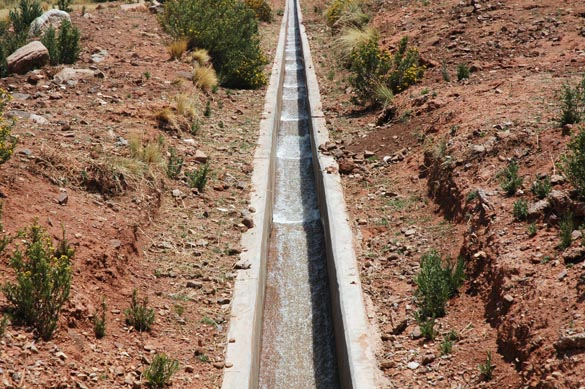Fig. 1   Roll waves in lateral canal, Cabana-Mañazo irrigation project, Puno, Peru.

The parameter β characterizes the type of friction and cross-sectional shape; for instance, β = 4/3 is applicable to Manning friction in a triangular channel. For a given type of friction and cross-sectional shape, the value of β is set. The neutral-stability Froude number Fns is that which corresponds to the Vedernikov number V = 1. The neutral-stability Froude number is a function only of β. From Eq. 10, the neutral-stability Froude number is:

 1   Fns =   ______                 β - 1
(11)

Table 1 shows values of Fns for selected values of β corresponding to typical combinations of types of friction and shape of cross section, from a high of β = 3 for laminar flow to a low of β = 1 for an inherently stable channel (Ponce 1991). To allow ready comparison, Col. 2 shows values of 24 β, to indicate the gradual decrease of β from 3 to 1.

 Table 1.  Values of Fns for selected values of β.      β 24 β Type of friction Shape of cross section Fns 3 72 Laminar Hydraulically wide 1/2 8/3 64 Mixed laminar-turbulent(25% turbulent Manning) Hydraulically wide 3/5 21/8 63 Mixed laminar-turbulent(25% turbulent Chezy) Hydraulically wide 8/13 7/3 56 Mixed laminar-turbulent(50% turbulent Manning) Hydraulically wide 3/4 9/4 54 Mixed laminar-turbulent(50% turbulent Chezy) Hydraulically wide 4/5 2 48 Mixed laminar-turbulent(75% turbulent Manning) Hydraulically wide 1 15/8 45 Mixed laminar-turbulent(75% turbulent Chezy) Hydraulically wide 8/7 5/3 40 Turbulent Manning Hydraulically wide 3/2 3/2 36 Turbulent Chezy Hydraulically wide 2 4/3 32 Turbulent Manning Triangular 3 5/4 30 Turbulent Chezy Triangular 4 1 24 Any Inherently stable ∞

Table 1 shows that the value β = 1 represents an asymptotic behavior, because for β  ⇒  1, the neutral-stability Froude number Fns  ⇒  ∞. Since the practical upper limit for the Froude number is much less than ∞, typically closer to 20 (Chow 1959), it follows that the condition β = 1 describes a channel that is always stable. A channel of rating such that β = 1 has been referred to as the inherently stable channel (Ponce 1991).

From Eq. 5, for β = 1, the flow velocity is a constant, α = Q/A. Thus, an inherently stable channel is one for which the flow velocity and hydraulic radius remain constant as the stage varies (Liggett 1975). The shape of the inherently stable channel has been documented by Ponce and Porras (1993) (Fig. 2).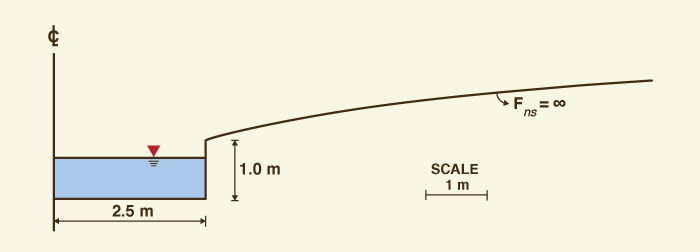Fig. 2   Cross-sectional shape of the inherently stable channel (Ponce and Porras 1993).

3.  VEN TE CHOW:  A SYNOPSIS

Ven Te Chow was born in Hangchow, China, on August 14, 1919. He received his B.S. in civil engineering from the National Chiao Tung University in 1940, his M.S. in engineering mechanics from Pennsylvania State University in 1948, and his Ph.D. in hydraulic engineering from the University of Illinois in 1950 (Ackermann 1984). He became a naturalized U.S. citizen in 1962, and was on the civil engineering faculty at the University of Illinois from 1948 until his death in 1981.

Chow is the author of the following widely acclaimed books: (1) Open-channel hydraulics, published in 1959, from which Fig. 3 was extracted; (2) Handbook of Applied Hydrology, published in 1964; and (3) Applied Hydrology, with coauthors David Maidment and Larry Mays, published in 1989.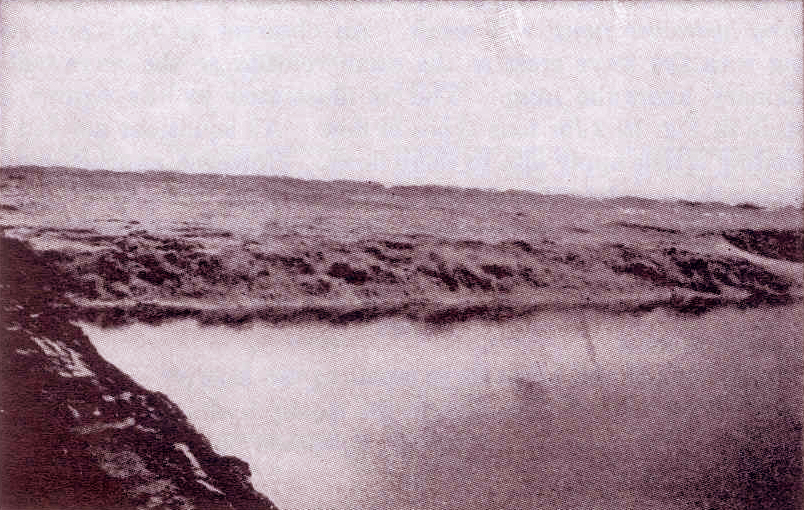Fig. 3   Tidal bore on the Chien Tang River, China (Chow 1959).

4.  THE FROUDE NUMBER

The Froude number is attributed to William Froude, who was born in Dartington, Devon, England in 1810, and died from a stroke on a cruise to South Africa in 1879, at age 69 (Fig. 4). In 1861, Froude wrote a paper on the design of ship stability in a seaway (http://froude.sdsu.edu). Later, between 1863 and 1867, working with physical models of ships, he showed that the frictional resistance in the model (at reduced scale) and prototype (the actual ship) were equal when the speed V was proportional to the length L to the power 1/2:

 V = k L1/2
(12)

in which k is a constant that applies to both model and prototype. Froude called this physical law the "Law of Comparison." He was the first to identify the most efficient shape for the hull of ships, as well as to predict ship stability based on studies using reduced-scale models.

In open-channel hydraulics, Froude's Law is embodied in the Froude number, defined as follows (Chow 1959; Brater and King 1976):

 V F  =    ________             (g D)1/2
(13)

in which D is the hydraulic depth, defined as the flow area divided by the top width. Note that for application to open-channel flow, the horizontal length L in Froude's Law (Eq. 12) has been replaced by the hydraulic depth D (Eq. 13). This equation is basically the same as Eq. 8, wherein the hydraulic depth D has been approximated as the flow depth y.Fig. 4   Portrait of William Froude (Wikipedia).

5.  THE VEDERNIKOV NUMBER

The concept of Vedernikov number was first published in a Soviet journal (Vedernikov 1945; 1946). Craya wrote about the same concept in a paper published in 1952 (Craya 1952). The Vedernikov-Craya criterion states that roll waves will form when the Seddon celerity equals or exceeds the Lagrange celerity, that is, when the kinematic wave celerity, governed by gravity and friction, exceeds the dynamic wave celerity, governed by the pressure gradient and inertia. This is the condition that the Vedernikov number V  ≥ 1.

Note the unfortunate confusion in Craya's seminal paper, where the Lagrange [dynamic wave] celerity is described as governed by gravity [sic] and inertia. The role of the several forces acting in unsteady flow in open channels (gravity, friction, pressure gradient, and inertia) has been clarified by Ponce and Simons (1977), who calculated the dimensionless relative wave celerity throughout the dimensionless wavenumber spectrum. Under Chezy friction, for Froude number F = 2, that is, V = 1, all waves propagate at the same celerity, regardless of size.

To reiterate, the Vedernikov-Craya criterion states that roll waves will form in an open channel under the following condition, in terms of absolute celerities:

 dQ                             ______   ≥   u   + ( g y )1/2      dA
(14)

In terms of relative celerities, roll waves will form when the relative Seddon celerity is greater than or equal to the relative Lagrange celerity:

 ( β - 1 ) u  ≥  ( g y )1/2
(15)

Figure 4 shows roll waves forming on the spillway of Turner reservoir, in San Diego County, California, following spillage after heavy rains, on February 24, 2005. Note that the no-slip condition on the vertical walls makes the waves appear as if the flow were three-dimensional.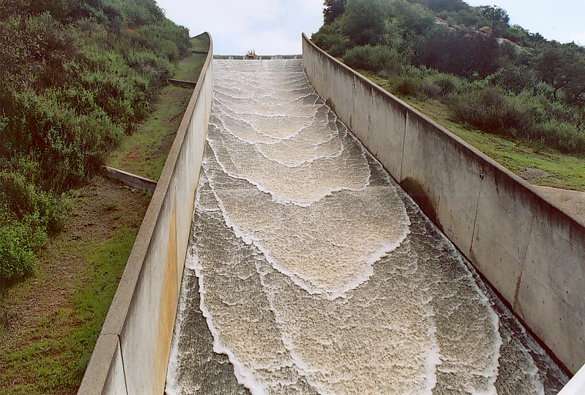Fig. 5   Roll waves on the spillway at Turner reservoir, San Diego County, California,on February 24, 2005.

6.  THE VEDERNIKOV NUMBER IN CHOW'S OPEN-CHANNEL HYDRAULICS

In Section 8-8, Instability of Uniform Flow, of Open-channel hydraulics, Ven Te Chow describes a criterion "which may be called the Vedernikov number" as follows:

 x γ u   V =   _________              ( g y )1/2
(16)

in which x = exponent of hydraulic radius R in the general velocity formula u = f(R), defined as follows:

 1 + b   x  =    ________                  2 - b
(17)

in which b = exponent of Reynolds number R in the frictional power law f = a R -b, in which f = Darcy-Weisbach friction factor. The value of b varies in the range 0-1, with b = 0 applicable to turbulent Chezy friction, b = 1/5 to turbulent Manning friction, and b = 1 to laminar flow. Thus, x = 1/2 for the Chezy formula, x = 2/3 for the Manning formula, and x = 2 for laminar flow.

The parameter γ is a cross-sectional shape factor defined as follows:

 dP   γ  =  1 -  R  _____                         dA
(18)

in which R = hydraulic radius; P = wetted perimeter; and A = flow area. The shape factor γ varies in the range 0-1. The value γ = 1 applies to a hydraulically wide channel, for which the wetted perimeter P is constant, and γ = 0 applies to an inherently stable channel, for which the hydraulic radius R is constant. To prove these assertions, define a power function relating wetted perimeter and flow area:

 P  =  k1 A d1
(19)

The derivative is:  dP/dA = d1 (P/A) = d1/R.

In Eq. 18, for γ = 1,  R (dP/dA) = 0; therefore: d1 = 0, and the wetted perimeter is constant equal to k1 and independent of the flow area A. Thus:  γ = 1 - d1. Conversely, for γ = 0, if follows that d1 = 1, and, given Eq. 19, the hydraulic radius A/P is constant equal to 1/k1 and independent of the flow area A.

With Eq. 8, Eq. 16 reduces to (Chow 1959):

 V  =   x γ F
(20)

which implies that the Vedernikov number is a function of the Froude number, a statement which is strictly not correct. The examination of Eqs. 8 to 10 reveals that the Froude and Vedernikov numbers are totally independent of each other. The confusion arises only circumstantially because the relative kinematic wave celerity v is expressed in terms of the mean velocity u (Eq. 6).

Given Eq. 10, it follows that:

 β  - 1  =   x γ
(21)

which states that β contains information on both friction (x) and cross-sectional shape (γ). Table 2 summarizes the relations between b, x, γ, d1 and β for a wide range of flow conditions.

 Table 2.  Variation of β, the exponent of the discharge-flow area rating.         Cross-sectionalshape Frictiontype b[In Eq. 17] x[Eq. 17] γ[Eq. 18] d1[In Eq. 19] β - 1[Eq. 21] β[In Eq. 4] Hydraulicallywide Laminar 1 2 1 0 2 3 Hydraulicallywide Manning 1/5 2/3 1 0 2/3 5/3 Chezy 0 1/2 1 0 1/2 3/2 Triangular Manning 1/5 2/3 1/2 1/2 1/3 4/3 Chezy 0 1/2 12 1/2 1/4 5/4 Inherentlystable Manning 1/5 2/3 0 1 0 1 Chezy 0 1/2 0 1 0 1

The question remains as to why Chow placed the Vedernikov number in Chapter 8 of his book, as the last section [Section 8-8] of the chapter entitled "Theoretical Concepts..." instead of placing it in Chapter 1, together with the Froude number and other fundamental concepts. This fact may have contributed to the relative obscurity of the Vedernikov number, which persists to this date despite the passing of more than half a century. Many practicing engineers, while they acknowledge having consulted the book many times, have yet to discover the Vedernikov number (Ponce 2003).

7.  CONCLUDING REMARKS

The concepts of Froude and Vedernikov numbers are reviewed on the occasion of the 50th anniversary of the publication of Ven Te Chow's Handbook of Applied Hydrology. While the Froude number (F) is standard fare in hydraulic engineering practice, the Vedernikov number (V) remains to be recognized by many practicing engineers. It is surmised here that this may be due in part to the fact that Chow placed the Vedernikov number in Chapter 8 of his book, instead of placing it in Chapter 1, together with the Froude number.

A comprehensive description of the variation of β, the altogether important exponent of the discharge-flow area rating = 1 + V/F), is accomplished here to recognize and honor the contributions of Professor Ven Te Chow to the hydraulic engineering profession.

REFERENCES

Ackermann, W. C. 1984. Ven Te Chow, 1919-1981. Memorial Tributes: National Academy of Engineering, Vol. 2.

Brater, E. F., and H. W. King. 1976. Handbook of Hydraulics. 6th Edition, McGraw-Hill, New York.

Chow, V. T. 1959. Open-channel hydraulics. McGraw-Hill, New York.

Craya, A. 1952. The criterion for the possibility of roll-wave formation. Gravity Waves, Circular 521, National Bureau of Standards, Washington, D.C., pages 141-151.

Liggett, J. A. 1975. Stability. Chapter 6 in Unsteady flow in open channels, Vol. 1, K. Mahmood and V. Yevjevich, editors, Water Resources Publications, pages 259-281.

Lighthill, M. J., and G. B. Whitham. 1955. On kinematic waves: I. Flood movement in long rivers. Proceedings of the Royal Society, Vol. 29, A, No. HY12, pages 281-316.

Ponce, V. M., and D. B. Simons. 1977. Shallow wave propagation in open channel flow. Journal of Hydraulic Engineering, ASCE, Vol. 103, No. HY12, pages 1461-1476, December.

Ponce, V. M. 1989. Engineering Hydrology: Principles and Practices. Prentice Hall, Englewood Cliffs, New Jersey.

Ponce, V. M. 1991. New perspective on the Vedernikov number. Water Resources Research, Vol. 27, No. 7, pages 1777-1779, July.

Ponce, V. M. 1992. Kinematic wave modelling: Where do we go from here? International Symposium on Hydrology of Mountainous Areas, Shimla, India, May 28-30, pages 485-495.

Ponce, V. M., and P. J. Porras. 1993. Effect of cross-sectional shape on free-surface instability. Journal of Hydraulic Engineering, ASCE, Vol. 121, No. 4, pages 376-380, April.

Ponce, V. M. 2003. That's the one we skip!  Legacy Tales, link 970 in ponce.sdsu.edu.

Powell, R. W. 1948. Vedernikov's criterion for ultra-rapid flow. Transactions, American Geophysical Union, Vol. 29, No. 6, 882-886.

Seddon, J. A. 1900. River hydraulics. Transactions, ASCE, Vol. XLIII, pages 179-243, June.

Vedernikov, V. V. 1945. Conditions at the front of a translation wave disturbing a steady motion of a real fluid. Comptes Rendus (Doklady) de l' Académie des Sciences de l' U.R.S.S., Vol. 48, No. 4, pages 239-242.

Vedernikov, V. V. 1946. Characteristic features of a liquid flow in an open channel. Comptes Rendus (Doklady) de l' Académie des Sciences de l' U.R.S.S., Vol. 52, No. 3, pages 207-210.

 191017